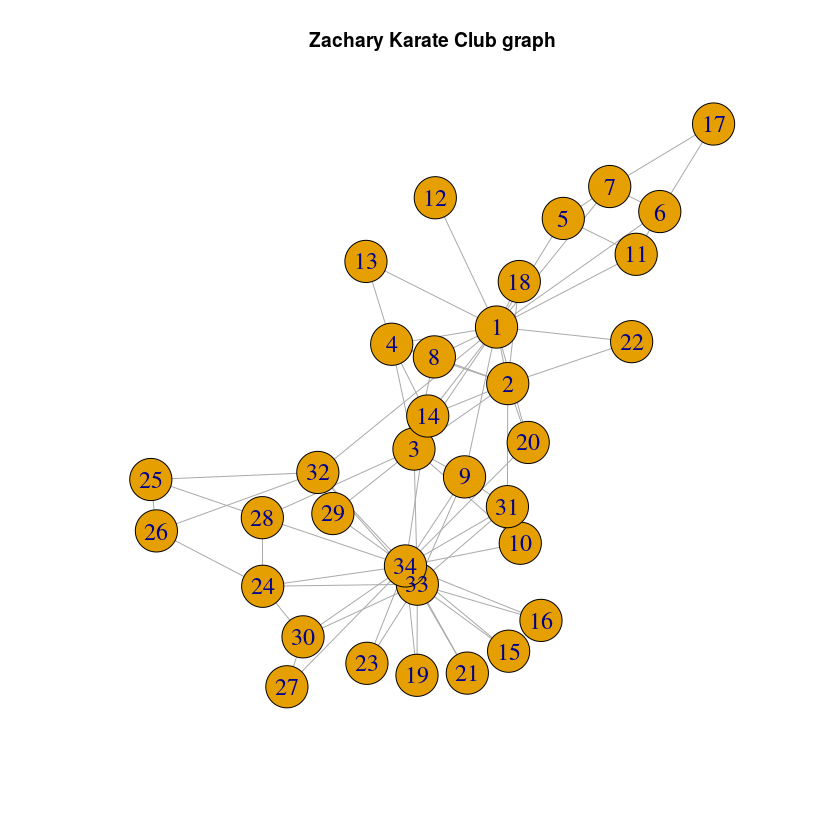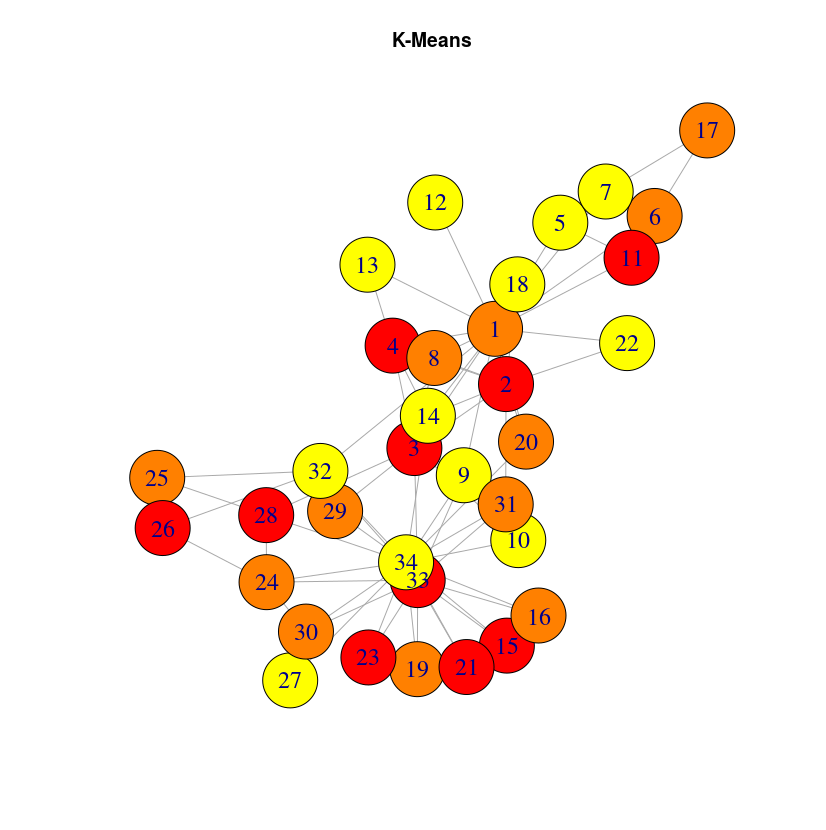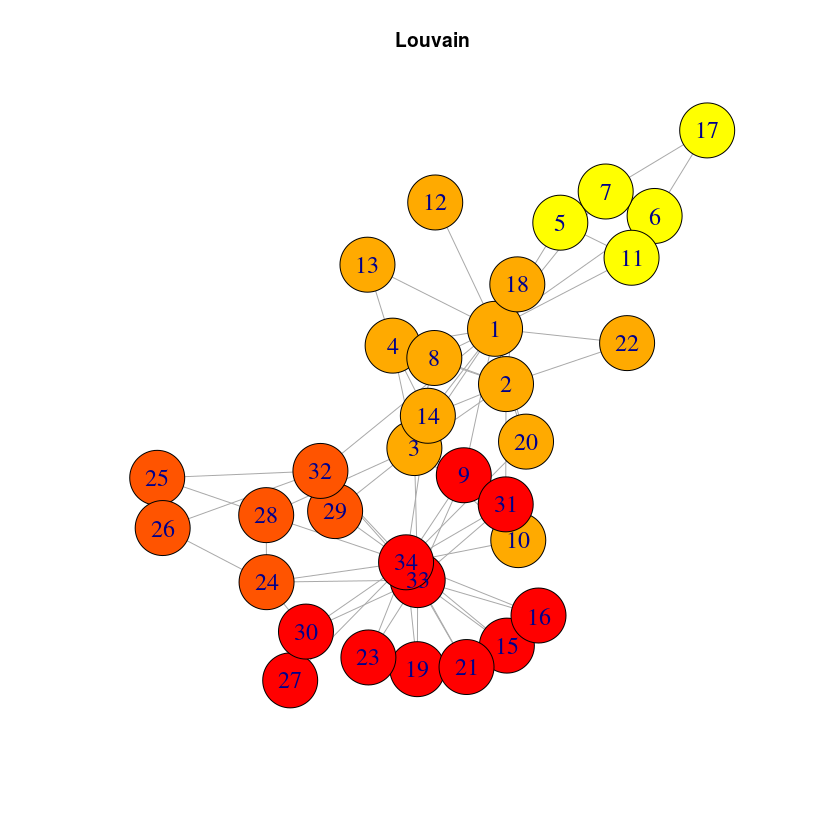# Deep Walk embedding on Zachary's Karate Club

## April 25, 2019

Graph embedding is a temendous topic, evolving very quickly. In this post, I want to show you how to use DeepWalk embedding on a Neo4j graph.

First, we will start from the famous Zachary’s karate club dataset. You can find the data and installation instructions here.

The graph looks like this:First, we will install the ml-models library for Neo4j. To do so, just download the last release jar from here and copy the jar file to the plugins directory of your database. If you are using Neo4j Desktop, you can easily find this folder by opening a terminal in the application or using the “Open Directory” tool in the management window.

Now you can start (or restart) your graph and we’ll directly use the deep walk embedding procedure. For explanations about how deep walk works, please check this article

So, let’s find the deep walk embedding for the ZKC graph. It uses the same pattern than the algorithms in the Neo4j graph algorithms library. We will use the procedure that writes the results into the graph:

CALL embedding.deepWalk()


At that step, you might see some errors related to security issues. In that case, you have to change your graph settings to modify this property:

dbms.security.procedures.unrestricted=*


and then restart neo4j.

Once the algorithm execution is working and finished, you can see that your nodes have a new property called deepWalk consisting in a list of numbers. Let’s extract this information into a csv file to analyze it:

MATCH (n)
RETURN n.pId, n.deepWalk


Let’s now import this data into a pandas.DataFrame :

import pandas as pd
d = d.set_index("n.pId")
# split the deepWalk string to columns
nd = d['n.deepWalk'].str.strip('[]').str.split(',').apply(pd.Series)


Now that we have dataframe where each row is a node and each column a feature learned by the DeepWalk algorithm, let’s run some clustering algorithm on it. But first, let’s run some PCA to reduce the number of features:

from sklearn.decomposition import PCA
from sklearn.cluster import KMeans
pca = PCA(n_components=2)
X_red = pca.fit_transform(nd)
kmeans = KMeans(n_clusters=3, random_state=1234)
kmeans.fit(X_red)
lab = kmeans.labels_


And finally plot the result:

plt.figure(figsize=(9 ,6))
plt.scatter(X_red[:,0], X_red[:,1], c=lab, s=300, cmap="prism", edgecolor="grey")

for i, txt in enumerate(d.index):
plt.annotate(txt, X_red[i],
horizontalalignment='center', verticalalignment='center',
)

plt.xlabel("PCA x1")
plt.ylabel("PCA x2")
plt.title("PCA decomposition from DeepWalk graph embedding \n + K-means clustering")
plt.grid()
plt.show()Let’s visualize the results in a different way, using the previous graph layout:It can be a bit surprising that close nodes do not belong to the same cluster. The reason is that DeepWalk algorithm will capture the role of the nodes, not their proximity. If you want to extract communities based on graph structure, you may want to use community detection algorithms like the Louvain algorithm, included into the graph algorithm library. Once this library is installed (very straightforward with Neo4j desktop, just check the plugins tab in your graph Manage window)

CALL  algo.louvain()


And you can check the result below: